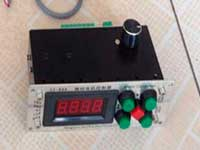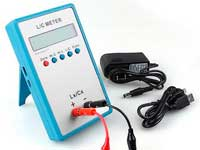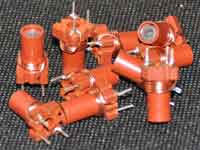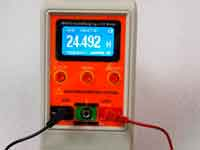Search coil for a metal detector - 5.0 out of 5 based on 1 vote
Rating 5.00 (1 Vote)

Search coil for a metal detectorMulti-turn loop of wire for metal detectors can be represented as a multi-layer air core coil with a square cross-section of winding. This coil belongs to the "Short coil class". Wheeler's simple formula is not suitable for calculating such a coil, as we noted in the article "Calculation of a multi-layer coil." So for metal detector coils we can use the universal algorithm for calculating a multi-layer coil, based on the Maxwell coaxial circular filament method. But since the cross section of the winding is square and the thickness of the coil is much smaller than its diameter, we can use a simplified calculation. For the first time, the formula for calculating such a coil was proposed by Maxwell:where:

• L - inductance (nano H);
• r - mean radius of the winding (cm);
• R - geometric mean distance of the wire bundle cross-section (cm);
• N - number of turns;Later in 1916, this formula was improved for more accurate calculations in the work of Rosa. A more understandable version of the Rosa's formula was published in 1931 by Brooks. See the web-links below. The Brooks formula is suitable to calculate the "Short" and "Medium" multilayer coils.
The Brooks formula was reduced to the modern form by George Overton in the book "Inside the Metal Detector".where:

• L - inductance (H);
• r - mean radius of the winding (half of the Dm) (m);
• l - length of the winding that is equal to the winding thickness T (m);
• N - number of turns;

The book has the calculation algorithm that is based on this formula, which we use in our open source Coil64 program with the kind consent of George Overton.
In the Coil32 program we use another variant of the same formula. So the results of the calculation by the both program are quite close.

References:

Сomments from anonymous guests are enabled with moderation.

Coil Winding MachineUS \$187.53
The system consists of individual components including a controller, a stepping motor, a foot switch, power adapter and output shaft. This design makes it very easy for you to integrate the system into your own project.

LC Meter LC200AUS \$37.99
Measuring Capacitance Range:
0.01 pF - 100mF
Measuring Inductance Range:
0.001 uH - 100H
Measurement accuracy:1%.

RF InductorsUS \$6.43
Variable Ferrite Core Inductor coil set 3.5Turns 25-100MHZ

Auto Ranging LCR MeterUS \$63.07
Meter can measure 0.00pF to 100.00mF capacitance, 0.000uH to 100.00H inductance and 0.00? to 10.000M? resistance. Very suitable for small value capacitor and inductor measurement.